# Finding the area of a trapezoid on a grid by using triangles and rectangles Online Quiz

#### Complete Python Prime Pack

9 Courses     2 eBooks

#### Artificial Intelligence & Machine Learning Prime Pack

6 Courses     1 eBooks

#### Java Prime Pack

9 Courses     2 eBooks

Following quiz provides Multiple Choice Questions (MCQs) related to Finding the area of a trapezoid on a grid by using triangles and rectangles. You will have to read all the given answers and click over the correct answer. If you are not sure about the answer then you can check the answer using Show Answer button. You can use Next Quiz button to check new set of questions in the quiz.Q 1 - Find the area of the following trapezoid by using triangles and rectangles.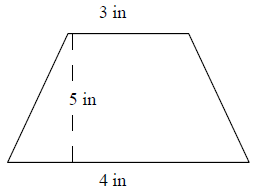### Explanation

Step 1:

Area of Right Triangle = $\frac{1}{2}$ × b × h; b = base; h = height.

Area of 2 right triangles = 2 × $\frac{1}{2}$ × b × h = 0.5 × 5 = 2.5 square in.

Step 2:

Area of Rectangle = l × w = 5 × 3 = 15 square in

Step 3:

Area of trapezoid = Area of triangles + Area of rectangle

= 2.5 + 15

= 17.5 square in

Q 2 - Find the area of the following trapezoid by using triangles and rectangles.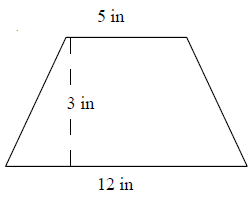### Explanation

Step 1:

Area of Right Triangle = $\frac{1}{2}$ × b × h; b = base; h = height.

Area of 2 right triangles = 2 × $\frac{1}{2}$ × b × h = 3.5 × 3 = 10.5 square in.

Step 2:

Area of Rectangle = l × w = 5 × 3 = 15 square in

Step 3:

Area of trapezoid = Area of triangles + Area of rectangle

= 10.5 + 15

= 25.5 square in

Q 3 - Find the area of the following trapezoid by using triangles and rectangles.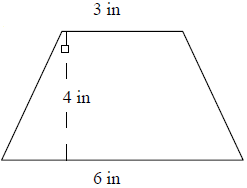### Explanation

Step 1:

Area of Right Triangle = $\frac{1}{2}$ × b × h; b = base; h = height.

Area of 2 right triangles = 2 × $\frac{1}{2}$ × b × h = 1.5 × 4 = 6 square in.

Step 2:

Area of Rectangle = l × w = 4 × 3 = 12 square in

Step 3:

Area of trapezoid = Area of triangles + Area of rectangle

= 6 + 12

= 18 square in

Q 4 - Find the area of the following trapezoid by using triangles and rectangles.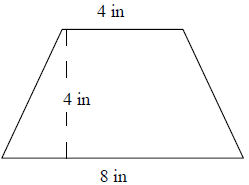### Explanation

Step 1:

Area of Right Triangle = $\frac{1}{2}$ × b × h; b = base; h = height.

Area of 2 right triangles = 2 × $\frac{1}{2}$ × b × h = 2 × 4 = 8 square in.

Step 2:

Area of Rectangle = l × w = 4 × 4 = 16 square in

Step 3:

Area of trapezoid = Area of triangles + Area of rectangle

= 8 + 16

= 24 square in

Q 5 - Find the area of the following trapezoid by using triangles and rectangles.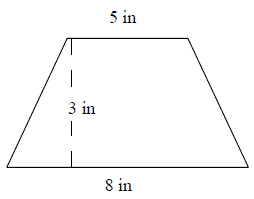### Explanation

Step 1:

Area of Right Triangle = $\frac{1}{2}$ × b × h; b = base; h = height.

Area of 2 right triangles = 2 × $\frac{1}{2}$ × b × h = 1.5 × 3 = 4.5 square in.

Step 2:

Area of Rectangle = l × w = 5 × 3 = 15 square in

Step 3:

Area of trapezoid = Area of triangles + Area of rectangle

= 4.5 + 15

= 19.5 square in

Q 6 - Find the area of the following trapezoid by using triangles and rectangles.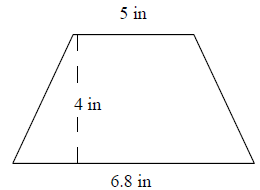### Explanation

Step 1:

Area of Right Triangle = $\frac{1}{2}$ × b × h; b = base; h = height.

Area of 2 right triangles = 2 × $\frac{1}{2}$ × b × h = 0.9 × 4 = 3.6 square in.

Step 2:

Area of Rectangle = l × w = 5 × 4 = 20 square in

Step 3:

Area of trapezoid = Area of triangles + Area of rectangle

= 3.6 + 20

= 23.6 square in

Q 7 - Find the area of the following trapezoid by using triangles and rectangles.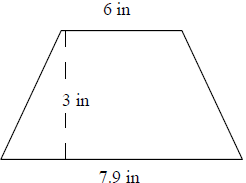### Explanation

Step 1:

Area of Right Triangle = $\frac{1}{2}$ × b × h; b = base; h = height.

Area of 2 right triangles = 2 × $\frac{1}{2}$ × b × h = 0.95 × 3 = 2.85 square in.

Step 2:

Area of Rectangle = l × w = 6 × 3 = 18 square in

Step 3:

Area of trapezoid = Area of triangles + Area of rectangle

= 2.85 + 18

= 20.85 square in

Q 8 - Find the area of the following trapezoid by using triangles and rectangles.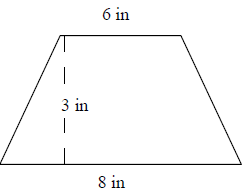### Explanation

Step 1:

Area of Right Triangle = $\frac{1}{2}$ × b × h; b = base; h = height.

Area of 2 right triangles = 2 × $\frac{1}{2}$ × b × h = 1 × 3 = 3 square in.

Step 2:

Area of Rectangle = l × w = 6 × 3 = 18 square in

Step 3:

Area of trapezoid = Area of triangles + Area of rectangle

= 3 + 18

= 21 square in

Q 9 - Find the area of the following trapezoid by using triangles and rectangles.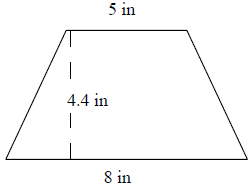### Explanation

Step 1:

Area of Right Triangle = $\frac{1}{2}$ × b × h; b = base; h = height.

Area of 2 right triangles = 2 × $\frac{1}{2}$ × b × h = 1.5 × 4.4 = 6.6 square in.

Step 2:

Area of Rectangle = l × w = 5 × 4.4 = 22 square in

Step 3:

Area of trapezoid = Area of triangles + Area of rectangle

= 6.6 + 22

= 28.6 square in

Q 10 - Find the area of the following trapezoid by using triangles and rectangles.### Explanation

Step 1:

Area of Right Triangle = $\frac{1}{2}$ × b × h; b = base; h = height.

Area of 2 right triangles = 2 × $\frac{1}{2}$ × b × h = 3.5 × 3.5 = 12.25 square in.

Step 2:

Area of Rectangle = l × w = 5 × 3.5 = 17.5 square in

Step 3:

Area of trapezoid = Area of triangles + Area of rectangle

= 12.25 + 17.5

= 29.75 square in

finding_area_of_trapezoid_on_grid_by_using_triangles_and_rectangles.htm